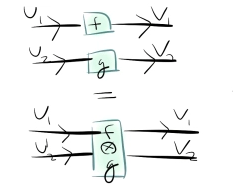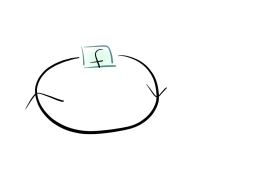jvns.ca Go back Open original

# Why are monoidal categories interesting?

Hello! Someone on Twitter asked a question about tensor categories recently and I remembered “oh, I know something about that!! These are a cool thing!“. Monoidal categories are also called “tensor categories” and I think that term is a little less confusing so I’m going to try to use that term in this post instead.

So here’s a quick stab at explaining why tensor categories are cool. I’m going to make a lot of oversimplifications which I figure is better than trying to explain category theory from the ground up. I’m not a category theorist (though I spent 2 years in grad school doing a bunch of category theory) and I will almost certainly say wrong things about category theory.

In this post I’m going to try to talk about Seven Sketches in Compositionality: An Invitation to Applied Category Theory using mostly plain English.

If you have been around functional programming for a bit, you might see the word “monoid” and “categories” and wonder “oh, is julia writing about monads, like in Haskell”? I am not!!

There is a sentence “monads are a monoid in the category of endofunctors” which includes both the word “monoid” and “category” but that is not what I am talking about at all. We’re not going to talk about types or Haskell or monads or anything.

### tensor categories are about proving (or defining) things with pictures

Here’s what I think is a really nice example from this “seven sketches in compositionality” PDF (on page 47):The idea here is that you have 3 inequalities

1. `t <= v + w`
2. `w + u <= x + z`
3. `v + x <= y`,

and you want to prove that `t + u <= y + z`.

You can do this algebraically pretty easily.

But in this diagram they’ve done something really different! They’ve sort of drawn the inequalities as boxes with lines coming out of them for each variable, and then you can see that you end up with a `t` and a `u` on the left and a `y` and a `z` on the right, and so maybe that means that `t + u <= y + z`.

The first time I saw something like this in a math class I felt like – what? what is happening? you can’t just draw PICTURES to prove things?!! And of course you can’t just draw pictures to prove things.

What’s actually happening in pictures like this is that when you put 2 things next to each other in the picture (like `t` and `u`), that actually represents the “tensor product” of `t` and `u`. In this case the “tensor product” is defined to be addition. And the tensor product (addition in this case) has some special properties –

1. it’s associative
2. if `a <= b` and `c <= d` then `a + c <= b + d`

so saying that this picture proves that `t + u <= y + z` actually means that you can read a proof off the diagram in a straightforward way:

`````` t + u <= (v + w) + u = v + (w + u) <= v + (x + z) = (v + x) + z <= y + z
``````

So all the things that “look like they would work” according to the picture actually do work in practice because our tensor product thing is associative and because addition works nicely with the `<=` relationship. The book explains all this in a lot more detail.

## draw vector spaces with “string diagrams”

Proving this simple inequality is kind of boring though! We want to do something more interesting, so let’s talk about vector spaces! Here’s a diagram that includes some vector spaces (U1, U2, V1, V2) and some functions (f,g) between them.Again, here what it means to have U1 stacked on top of U2 is that we’re taking a tensor product of U1 and U2. And the tensor product is associative, so there’s no ambiguity if we stack 3 or 4 vector spaces together!

This is all explained in a lot more detail in this nice blog post called introduction to string diagrams (which I took that picture from).

## define the trace of a matrix with a picture

So far this is pretty boring! But in a follow up blog post, they talk about something more outrageous: you can (using vector space duality) take the lines in one of these diagrams and move them backwards and make loops. So that lets us define the trace of a function `f : V -> V` like this:This is a really outrageous thing! We’ve said, hey, we have a function and we want to get a number in return right? Okay, let’s just… draw a circle around it so that there are no lines left coming out of it, and then that will be a number! That seems a lot more natural and prettier than the usual way of defining the trace of a matrix (“sum up the numbers on the diagonal”)!

When I first saw this I thought it was super cool that just drawing a circle is actually a legitimate way of defining a mathematical concept!

## what does this have to do with programming?

Even though this is usually a programming blog I don’t know whether this particular thing really has anything to do with programming, I just remembered I thought it was cool. I wrote my master’s thesis (which i will link to even though it’s not very readable) on topological quantum computing which involves a bunch of monoidal categories.

Some of the diagrams in this post are sort of why I got interested in that area in the first place – I thought it was really cool that you could formally define / prove things with pictures. And useful things, like the trace of a matrix!